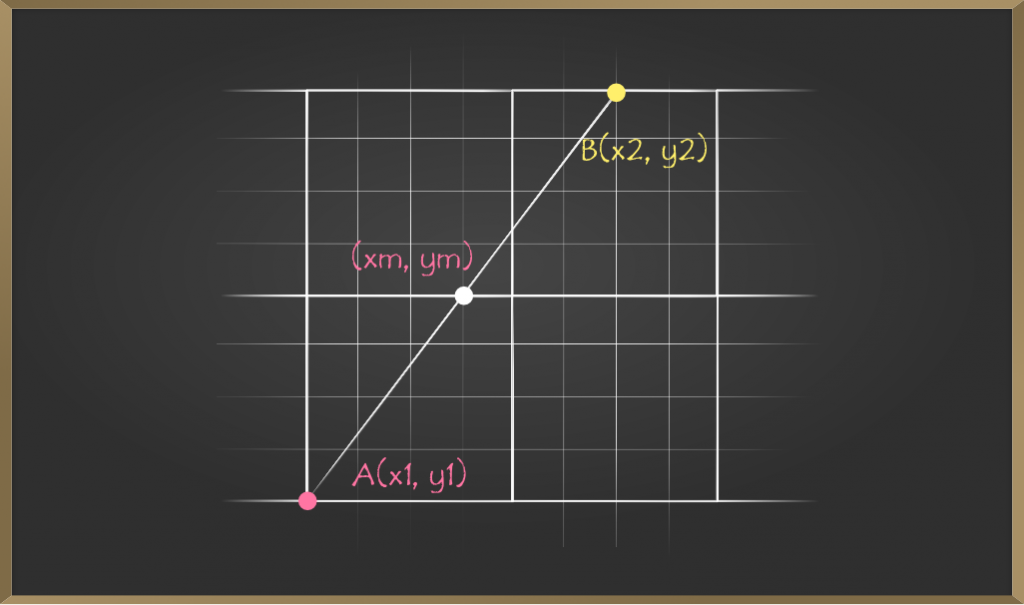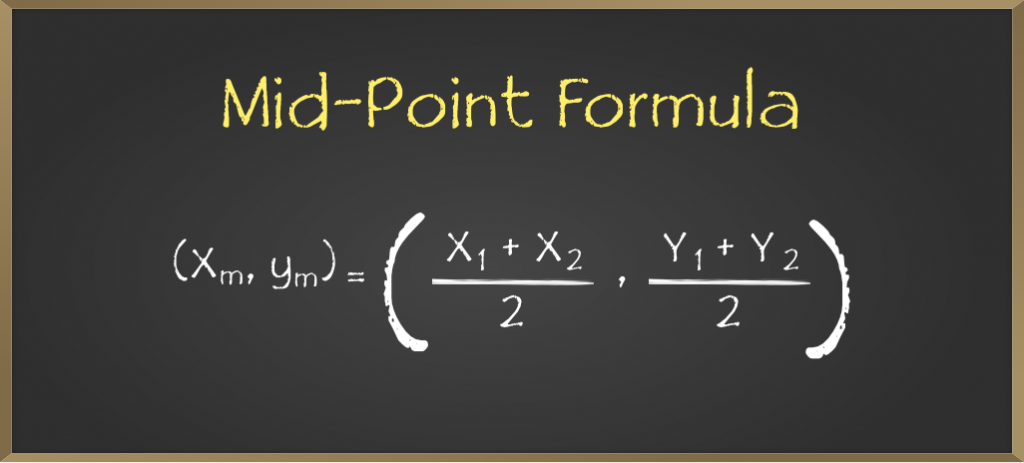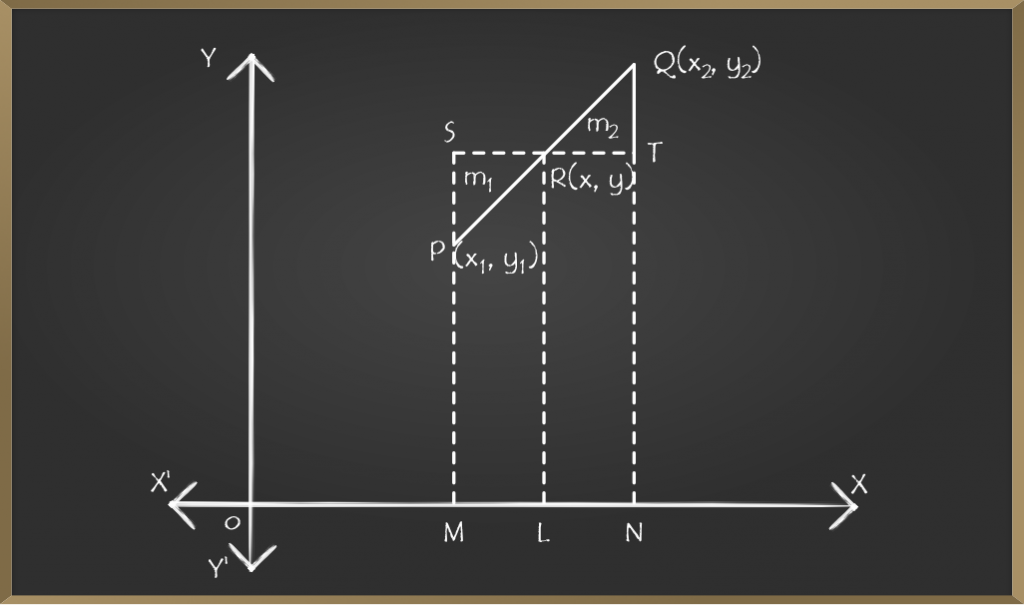# Mid-point Formula in Coordinate Geometry

• Difficulty Level : Medium
• Last Updated : 26 Oct, 2020

In geometry, a mid-point is the middle point of a line segment which is equidistant from both the endpoints. That point bisects the line into two equal halves. There are instances in Coordinate Geometry that we need to know the mid-point of two given points or mid-point of a line segment. In a cartesian plane, the midpoint of a line has its x-value as halfway between x-values of both endpoints and its y-value as halfway between y-values of both endpoints.For a line segment AB in Cartesian coordinate where the x-axis coordinate of point A is x1 and the y-axis coordinate of point A is y1 and similarly the x-axis coordinate of the point B is x2 and the y-axis coordinate of the point B is y2, the mid-point of the line will be given by (xm,ym).

The formula for mid-point  (xm,ym) is,## Derivation of Formula

Let P(x1,y1) and Q(x2,y2) be the two ends of a given line in a coordinate plane, and R(x,y) be the point on that line which divides PQ in the ratio m1:m2 such that

`PR/RQ = m1/m2              ...(1)`Drawing lines PM, QN, and RL perpendicular on the x-axis and through R draw a straight line parallel to the x-axis to meet MP at S and NQ at T.

Hence from the figure, we can say,

```SR = ML = OL - OM = x - x1            ...(2)
RT = LN = ON - Ol = x2 - x            ...(3)
PS = MS - MP = LR - MP = y - y1       ...(4)
TQ = NQ - NT = NQ - LR = y2 - y       ...(5)```

Now triangle SPR is similar to triangle TQR,

Therefore,

`SR/RT = PR/RQ`

By using equation 2, 3, and 1, we know,

```x - x1 / x2 - x = m1 / m2
m2x - m2x1 = m1x2 - m1x
m1x + m2x = m1x2 + m2x1
(m1 + m2)x = m1x2 + m2x1
x = (m1x2 + m2x1) / (m1 + m2)```

Now triangle SPR is similar to triangle TQR,

Therefore,

`PS/TQ = PR/RQ`

By using equation 4, 5, and 1, we know,

```y - y1 / y2 - y = m1 / m2
m2y - m2y1 = m1y2 - m1y
m1y + m2y = m1y2 + m2y1
(m1 + m2)y = m1y2 + m2y1
y = (m1y2 + m2y1) / (m1 + m2)```

Hence the coordinates of R(x,y) are,

`R(x, y)= (m1x2 + m2x1) / (m1 + m2), (m1y2 + m2y1) / (m1 + m2)`

As we had to calculate the mid point therefore we keep the values both of m1 and m2 as same i.e.

For mid-point,

`m1 = m2 = 1`

Hence,

`x, y = (1.x2 + 1.x1) / (1 + 1), (1.y2 + 1.y1) / (1 + 1)`

x, y = (x2 + x1) / 2, (y2 + y1) / 2

## Sample Problems on Mid-Point Formula

Example 1: What is the mid-point of the line segment AB where point A is at (6,8) and point B is (3,1)?

Solution: Let the midpoint be M(xm,ym),

xm = (x1 + x2) / 2

x1 = 6, x2 = 3

xm = (6 + 3) / 2 = 9 / 2 = 4.5

ym = (y1 + y2) / 2

y1 = 8, y2 = 1

ym = (8 + 1) / 2 = 9 / 2 = 4.5

Hence the midpoint of line AB is (4.5, 4.5).

Example 2: What is the mid-point of the line segment AB where point A is at (-6,4) and point B is (4,2)?

Solution: Let the midpoint be M(xm,ym),

x1 = -6, x2 = 4, y1 = 4, y2 = 2

(xm, ym) = ((x1 + x2) / 2, (y1 + y2) / 2)

(xm, ym) = ((-6 + 4) / 2, (4 + 2) / 2)

(xm, ym) = ((-2) / 2, (6) / 2)

(xm, ym) = (-1, 3)

Hence the midpoint of line AB is (-1, 3).

Example 3: Find the value of p so that (–2, 2.5) is the midpoint between (p, 2) and (–1, 3).

Solution: Let the midpoint be M(xm, ym) = (-2, 2.5) where,

x1 = -1, xm = -2

y-coordinate of the end point is already known as 2, hence we need to find only the x-coordinate

xm = (x1 + x2) / 2

-2 = (-1 + p) / 2

-4 = -1 + p

p = -3

Hence the other end-point of the line is (-3, 2).

My Personal Notes arrow_drop_up